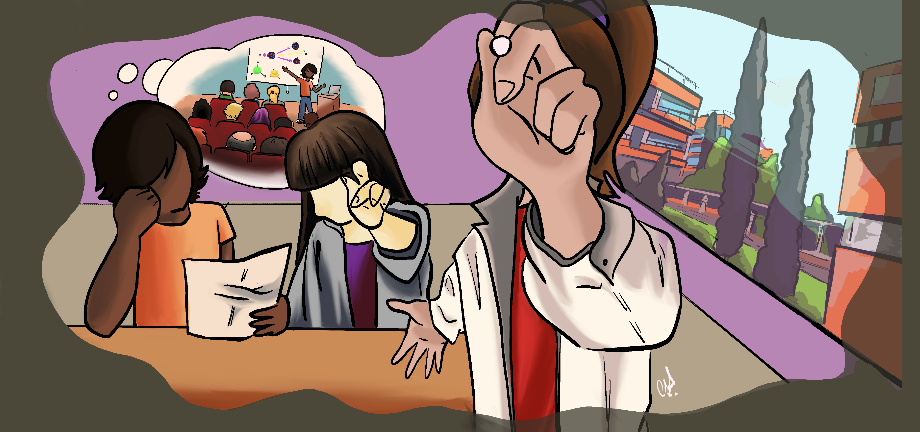#@FlipPhysics

21-25 March 2022
Salon de actos del IATA

## Study of $\alpha-$transfer reactions with $^7$Be in the context of nuclear astrophysics

Not scheduled
15m
Salon de actos del IATA

#### Salon de actos del IATA

Carrer del Catedràtic Agustín Escardino Benlloch, 7, 46980 Paterna, Valencia
Talk

### Speaker

Kabita Kundalia (Bose Institute, India)

### Description

In stellar evolution, the rate of $^{12}$C($\alpha$,$\gamma$)$^{16}$O reaction controls the C/O abundance ratio at the end of the helium burning phase, thus defining the further course of development. At stellar temperatures of around 300 keV, the cross section of $^{12}$C($\alpha$,$\gamma$)$^{16}$O is $\sim$ 10$^{-17}$ b, which cannot be measured using current technology. The $\alpha-$capture reaction populating the natural-parity states of the residual nuclei, is an effective indirect tool for studying these types of reactions. In this case, it corresponds to the alpha pickup by $^{12}$C to populate states of $^{16}$O, predominantly the 6.917 MeV state. Loosely bound stable nuclei with prominent $\alpha-$cluster structure, such as $^{6,7}$Li, $^{11}$B have also been used in such studies provided that these are "direct" $\alpha-$transfer and do not proceed via a compound nucleus. However, the breakup contributions from such nuclei have a significant impact on the transfer channels. Interestingly, the $^7$Be nucleus, though having an $\alpha-$cluster structure and a lower breakup threshold of 1.58 MeV, demonstrates lower breakup contribution compared to transfer cross section. In this context, we carried out an experiment at HIE-ISOLDE, CERN, with $^7$Be + $^{12}$C at E = 5 MeV/A to study $\alpha-$transfer reactions populating states in $^{16}$O, that dominantly contribute to the He-burning process. Preliminary results would be presented.

### Primary author

Kabita Kundalia (Bose Institute, India)

### Co-authors

Prof. Dhruba Gupta (Bose Institute, India) Mr. Sk Mustak Ali (Bose Institute) Prof. Swapan K Saha (Bose Institute, Former Faculty) Olof Tengblad (IEM -CSIC) Javier Díaz Ovejas (IEM-CSIC) Angel Perea Martinez (IEM/CSIC) Ismael Martel (Univesity of Huelva) Prof. Joakim Cederkall (Lund University, Sweden) Dr. Joochun Park (Institute for Basic Science, South Korea) Dr. Stuart Szwec (University of Jyvaskyla)

### Presentation Materials

There are no materials yet.
###### Your browser is out of date!

Update your browser to view this website correctly. Update my browser now

×